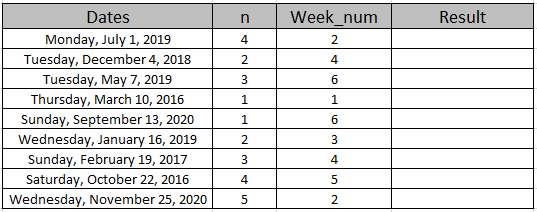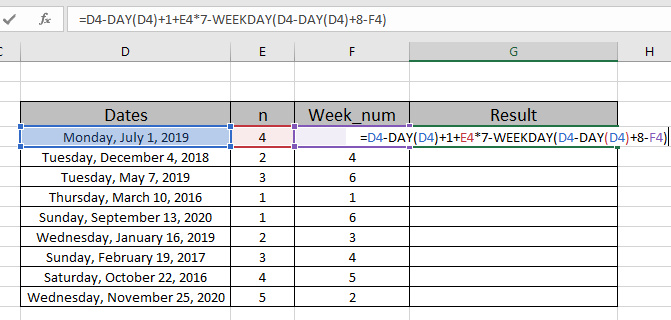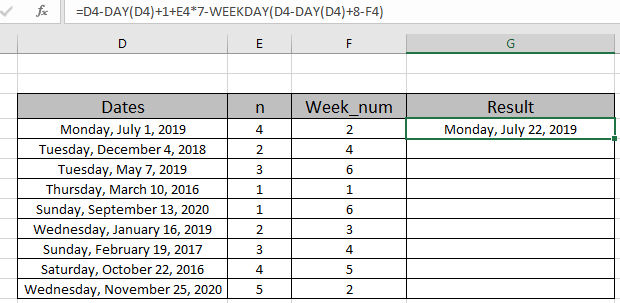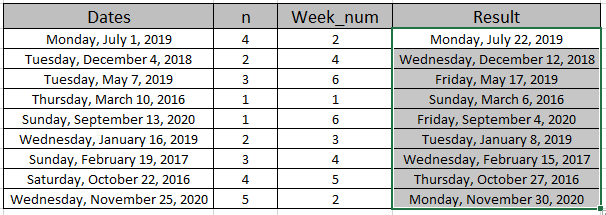# Nth weekday of month in Excel

In this article, we will learn how to get Nth weekday of month in Excel.

At Instance, while working with date values, sometimes we need to get the 2nd Wednesday of September given any date of the month in Excel.
How to solve the problem?

For this article we will be required to use the DAY function & WEEKDAY function. Now we will make a formula out of these functions. Here we are given dates of month and n value and specific weekday number. We need to get the nth weekday of the month.

Generic formula:

= date - DAY (date) + 1 + n * 7 - WEEKDAY (date - DAY (date) + 8 - Week_num)

Week_num : number representing the weekday of the week. Starts from Sunday as 1 and goes on to Saturday as 7.

Example:

All of these might be confusing to understand. So, let's test this formula via running it on the example shown below.

Here we have some date records and we need to find the nth weekday date values where n and Week_num givenNow we will use the following formula to get the 4th Monday of July
Use the Formula:

= D4 - DAY (D4) + 1 + E4 * 7 - WEEKDAY (D4 - DAY (D4) + 8 - F4 )

D4 : cell reference for date value

E4 : cell reference for n value

F4 :  cell reference for Week_num

Explanation:

• DAY(D4) returns 1 as value as shown below.

= D4 - 1 + 1 + 4*7 - WEEKDAY ( D4 - 1 + 8 - 2 )

• DAY (D4) + 1 + E4 * 7 returns 30 as result.
• So the date value when subtracted from 30 gives last month's date.
• WEEKDAY (D4 - DAY (D4) + 8 - F4 returns 7 as result.Here the year value is given as cell reference. Press Enter to get the count.As you can see the output date is 4th Monday of the month. Now copy and paste the formula to other cells using the CTRL + D or drag down option of Excel.As you can see in the above snapshot we obtain all the date values satisfying the n value and week_num using the Excel formula.

Here are some observational notes shown below.

Notes:

1. The formula only works with date values.
2. Date as argument to the function be given as cell reference or else the formula returns error.
3. Change the format of the cell to long date format using the Ctrl + 1 or select the format cell option from Home > Number option.
4. The argument array must be of the same length else the function.

Hope this article about how to Nth weekday of month in Excel is explanatory. Find more articles on Excel Date & Time functions here.

If you liked our blogs, share it with your friends on Facebook. And also you can follow us on Twitter and Facebook. We would love to hear from you, do let us know how we can improve, complement or innovate our work and make it better for you. Write us at info@exceltip.com

Related Articles

How to use the EDATE function in Excel

Calculate age from date of birth

Calculate Weighted Average

How to Group Data By Age Range in Excel

Get day name from Date in Excel

Get Month name from Date in Excel

How to use wildcards in excel

Popular Articles

50 Excel Shortcut to Increase Your Productivity

Edit a dropdown list

Absolute reference in Excel

If with conditional formatting

If with wildcards

Vlookup by date

Convert Inches To Feet and Inches in Excel 2016

Join first and last name in excel

Count cells which match either A or B

Terms and Conditions of use

The applications/code on this site are distributed as is and without warranties or liability. In no event shall the owner of the copyrights, or the authors of the applications/code be liable for any loss of profit, any problems or any damage resulting from the use or evaluation of the applications/code.